# Scientific Explanation Suppose we assume that explanation and

• Slides: 7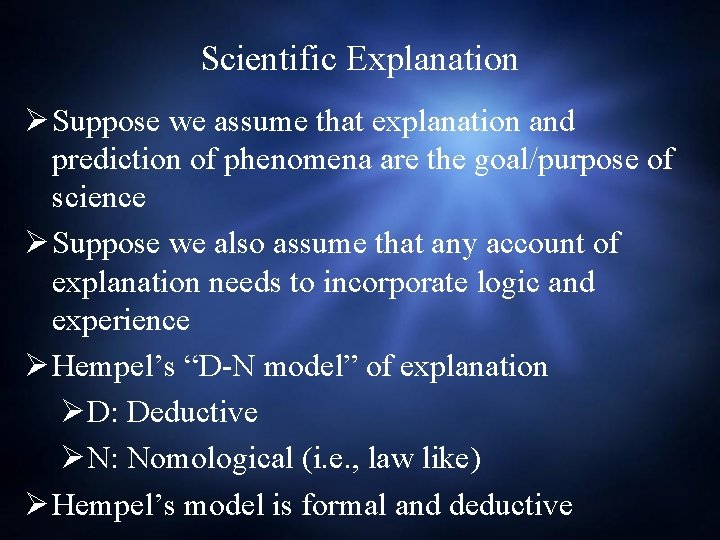Scientific Explanation Ø Suppose we assume that explanation and prediction of phenomena are the goal/purpose of science Ø Suppose we also assume that any account of explanation needs to incorporate logic and experience Ø Hempel’s “D-N model” of explanation ØD: Deductive ØN: Nomological (i. e. , law like) Ø Hempel’s model is formal and deductive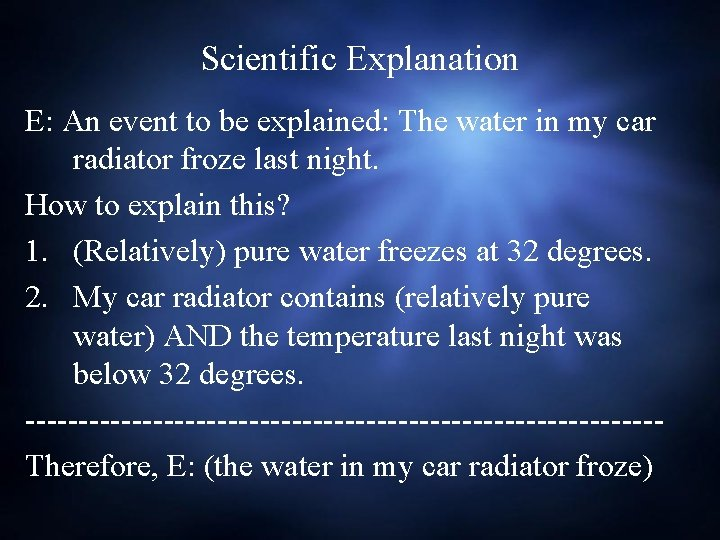Scientific Explanation E: An event to be explained: The water in my car radiator froze last night. How to explain this? 1. (Relatively) pure water freezes at 32 degrees. 2. My car radiator contains (relatively pure water) AND the temperature last night was below 32 degrees. ------------------------------Therefore, E: (the water in my car radiator froze)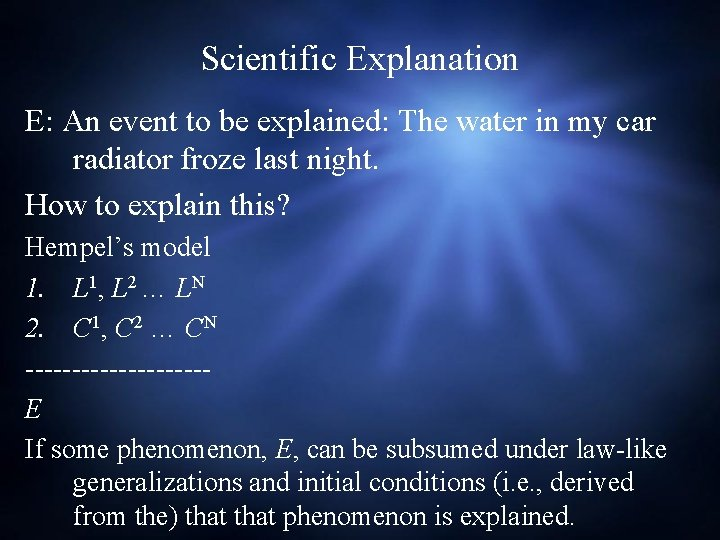Scientific Explanation E: An event to be explained: The water in my car radiator froze last night. How to explain this? Hempel’s model 1. L 1, L 2 … LN 2. C 1, C 2 … CN ----------E If some phenomenon, E, can be subsumed under law-like generalizations and initial conditions (i. e. , derived from the) that phenomenon is explained.Scientific Prediction E: An event predicted: The water in my car radiator will freeze last night. Hempel’s model 1. (Relatively) pure water freezes at 32 degrees (L) 2. My car radiator contains relatively free water and the temperature tonight will go below less than 32 degrees (C 1 and C 2). ----------------------------------So, the water is my car radiator will freeze tonight (E).Scientific Explanations and Predictions Ø Challenges to Hempel’s model of explanation: Ø What about sciences (such as many fields in biology) that do not have law-like statements? Are they not able to offer explanations? Ø Hempel: Some sciences include probabilistic generalizations from which probable explanations and/or predictions do follow. Ø The problem of asymmetry: examples in which while two statements can be derived from each other, only one way of construing their relationships is reasonably causal.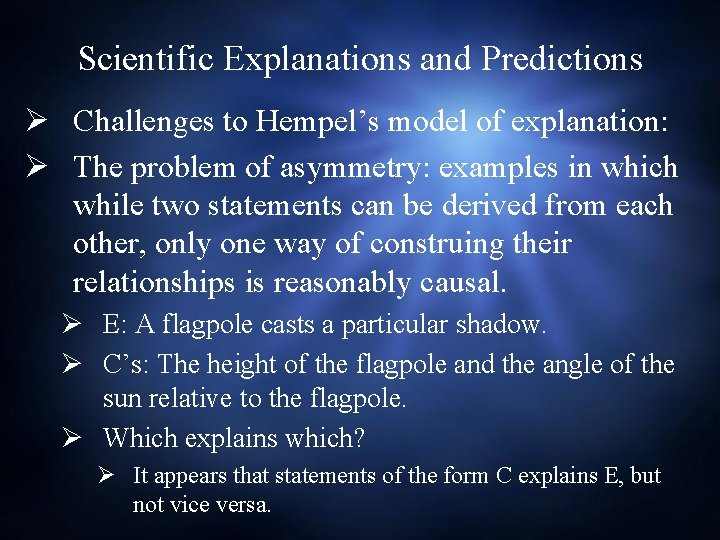Scientific Explanations and Predictions Ø Challenges to Hempel’s model of explanation: Ø The problem of asymmetry: examples in which while two statements can be derived from each other, only one way of construing their relationships is reasonably causal. Ø E: A flagpole casts a particular shadow. Ø C’s: The height of the flagpole and the angle of the sun relative to the flagpole. Ø Which explains which? Ø It appears that statements of the form C explains E, but not vice versa.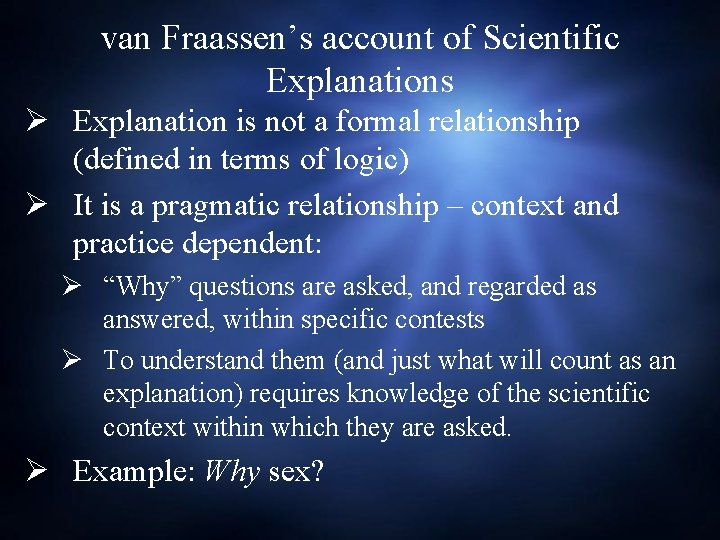van Fraassen’s account of Scientific Explanations Ø Explanation is not a formal relationship (defined in terms of logic) Ø It is a pragmatic relationship – context and practice dependent: Ø “Why” questions are asked, and regarded as answered, within specific contests Ø To understand them (and just what will count as an explanation) requires knowledge of the scientific context within which they are asked. Ø Example: Why sex?